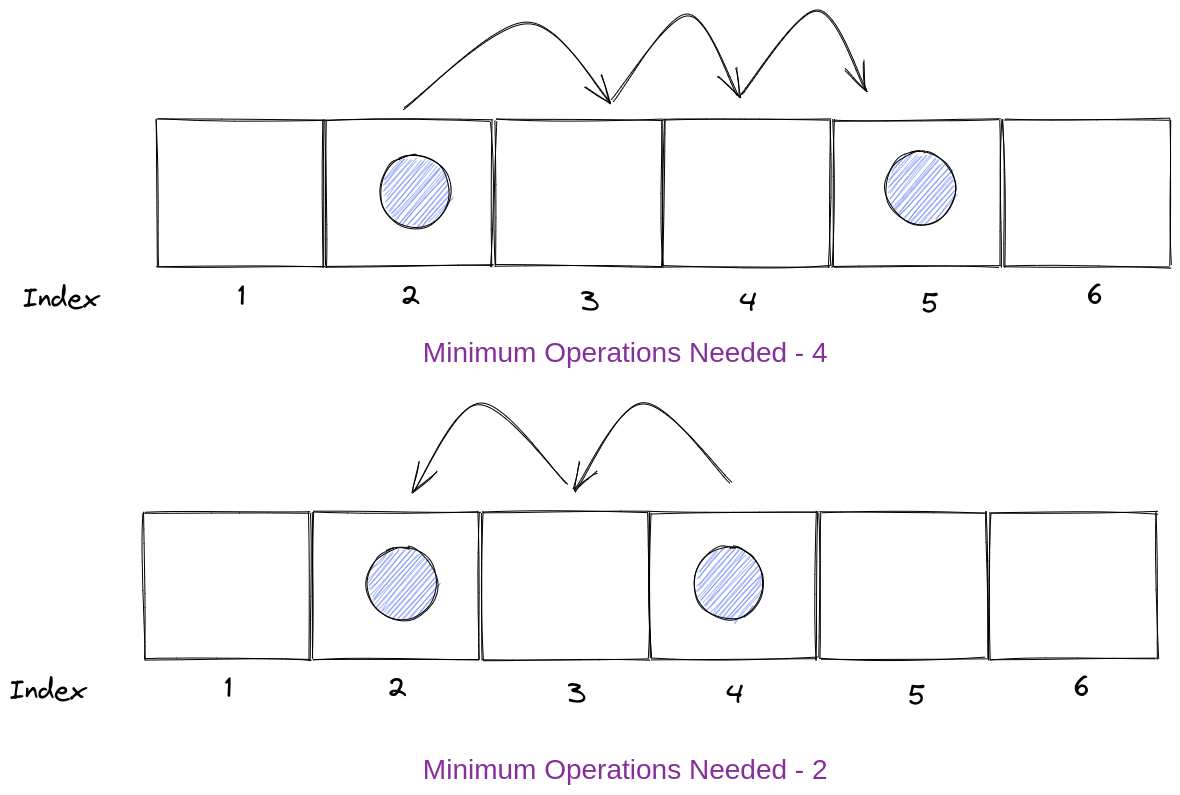Pankaj Tanwar
Published on

# Minimum Number of Operations to Move All Balls to Each Box.

Authors
•Name
Pankaj Tanwar
@the2ndfloorguy

Hello people, it's day #14. And enough of easy problems, let's try a medium one today.

TLDR;

• A really really interesting problem to solve, does justice to the medium tag
• Hopefully, After spending ~30 minutes, I was able to come up with the most optimized approach (without looking at the solution)

Problem of the day - Minimum Number of Operations to Move All Balls to Each Box

Tag - Medium

You have n boxes. You are given a binary string boxes of length n, where boxes[i] is '0' if the ith box is empty, and '1' if it contains one ball.

In one operation, you can move one ball from a box to an adjacent box. Box i is adjacent to box j if abs(i - j) == 1. Note that after doing so, there may be more than one ball in some boxes.

Return an array answer of size n, where answer[i] is the minimum number of operations needed to move all the balls to the ith box.

Each answer[i] is calculated considering the initial state of the boxes.

Example 1:

Input: boxes = "110" Output: [1,1,3] Explanation: The answer for each box is as follows:

1. First box: you will have to move one ball from the second box to the first box in one operation.
2. Second box: you will have to move one ball from the first box to the second box in one operation.
3. Third box: you will have to move one ball from the first box to the third box in two operations, and move one ball from the second box to the third box in one operation.

Problem statement and explanation of sample test was pretty straight forward and easy to understand.

So, for minimum operations to move a ball from one index to another index is absolute difference of both indexes. As from below image, you can see to move a ball from index 1 to 5, minimum operations required are 4. Same way, to move a ball from index 4 to 2, minimum operations required are 2.My first approach (and the worst & slowest one) was to use nested loop to get minimum operations for each index.

Here is the code -

class Solution {public:    vector<int> minOperations(string boxes) {        int total_box = boxes.length();        vector<int> ans;        int total = 0;        for(int box=0; box < total_box; box++) {            total = 0;            for(int box_index=0;box_index < total_box;box_index++) {                total += boxes[box_index] == '1' ? abs(box-box_index) : 0;            }            ans.push_back(total);        }        return ans;    }};

I soon realized, it is pretty bad and slow. Leetcode compiler confirmed it, 340ms, only faster than 7% of submissions. Oops. I started thinking of some pattern or some way to avoid nested loops here.

After a keep observation, I observed, If I know the minimum operations needed to move all balls from their respective side, for both, left and right side. I can calculate the result for current index.

Ahh, I know that was the most complex sentence in the world. Let's use some sweet pictures to visualize it and understand it clearly.

Suppose, I want to calculate minimum operations to move all balls, which are in the left side of index 2. For that, I can just check, how many operations were needed for left balls for index 1, how many balls were there and left index has any ball or not. Total of it from both side, left and right will give us the result.

One line logic - First, we move ball from left to right to track, how many ops it takes to for each box. Repeat the same process from right to left and return the total.

Here is my code -

class Solution {public:    vector<int> minOperations(string boxes) {        vector<int> res(boxes.length(), 0);                int count_of_one = 0;        int curr_val = 0;        bool prev_item_was_one = false;                // front iteration        for(int box=0; box<boxes.length(); box++) {            prev_item_was_one = (box > 0 && boxes[box-1] == '1');                        curr_val = curr_val + count_of_one + (prev_item_was_one ? 1 : 0);            count_of_one += (prev_item_was_one ? 1 : 0);                        res[box] = curr_val;        }                      count_of_one = 0;        curr_val = 0;        prev_item_was_one = false;                // back iteration        for(int box=boxes.length()-1; box>=0; box--) {            prev_item_was_one = (box < boxes.length()-1 && boxes[box+1] == '1');                        curr_val = curr_val + count_of_one + (prev_item_was_one ? 1 : 0);            count_of_one += (prev_item_was_one ? 1 : 0);                        res[box] += curr_val;        }            return res;    }};

I was very much confident about my code. Boom, runtime from 340ms to just 8ms, faster then 82% submissions. As you can see, time complexity is o(n) now.

It was a really nice problem to solve. Figuring out the pattern is really important which I think comes from practice only.

You might like previous editions of my coding diary